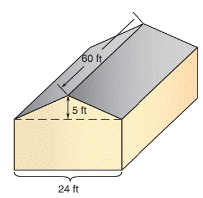Chapter 8.1, Problem 26EElementary Geometry For College St...

7th Edition
Alexander + 2 others
ISBN: 9781337614085

Solutions

Chapter
SectionElementary Geometry For College St...

7th Edition
Alexander + 2 others
ISBN: 9781337614085
Textbook Problem

The roof of the house shown needs to be reshingled.a) Considering that the front and back sections of the roof have equal areas, find the total area to be reshingled.b) If roofing is sold in squares (each covering 100  ft 2 ), how many squares are needed to complete the work?c) To remove old shingles and replace with new shingles costs \$97.50 per square. What is the cost of reroofing?To determine

a)

To find:

The total area of the roof of the house to be reshingled.

Explanation

Finding the area of the figure is followed by identifying the figure and find area with the suitable geometry formulae.

Calculation:

Given,

The total area of the roof(A)=(A1)Area of front roof+(A2)area of back roof

Front and back sections of the roof have equal areas.

Here,

A1=A2

A=A1+A1

A=2A1

Length of the rectangular

Front roof is l=60ft and by the figure BC is the width (w) of the rectangular front roof

Find width of the rectangular front roof:

For triangle ABC,

BD is the median of the AC.

Where,

(i.e

To determine

b)

To find:

The number of squares are needed to complete the work.

To determine

c)

To find:

The cost of reroofing.

Still sussing out bartleby?

Check out a sample textbook solution.

See a sample solution

The Solution to Your Study Problems

Bartleby provides explanations to thousands of textbook problems written by our experts, many with advanced degrees!

Get Started

23. Use class marks to find the mean, mode, and median of the data in Problem 7.

Mathematical Applications for the Management, Life, and Social Sciences

What does the Theorem of Pappus say?

Single Variable Calculus: Early Transcendentals

The radius of convergence of is: 1 3 ∞

Study Guide for Stewart's Multivariable Calculus, 8th

limxx2+13x+2= a) 0 b) 1 c) 13 d) does not exist

Study Guide for Stewart's Single Variable Calculus: Early Transcendentals, 8th